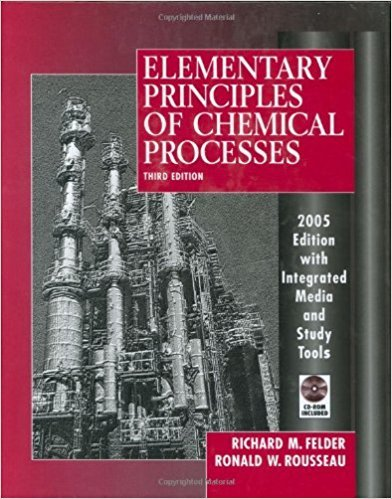×
Get Full Access to Elementary Principles Of Chemical Processes - 3 Edition - Chapter 11 - Problem 11.3
Get Full Access to Elementary Principles Of Chemical Processes - 3 Edition - Chapter 11 - Problem 11.3

×

# Methanol is added to a storage tank at a rate of 1200 kg/hISBN: 9780471687573 143

## Solution for problem 11.3 Chapter 11

Elementary Principles of Chemical Processes | 3rd Edition

• Textbook Solutions
• 2901 Step-by-step solutions solved by professors and subject experts
• Get 24/7 help from StudySoup virtual teaching assistantsElementary Principles of Chemical Processes | 3rd Edition

4 5 1 351 Reviews
24
2
Problem 11.3

Methanol is added to a storage tank at a rate of 1200 kg/h and is simultaneously withdrawn at a rate mw(t) (kg/h) that increases linearly with time. At t = 0 the tank contains 750 kg of the liquid and mw = 750 kg/h. Five hours later mw equals 1000 kg/h. (a) Calculate an expression for mw(t), letting t = 0 signify the time at which mw = 750 kg/h, and incorporate it into a differential methanol balance, letting M (kg) be the mass of methanol in the tank at any time. (b) Integrate the balance equation to obtain an expression for M(t) and check the solution two ways. (See Example 11.2-1.) For now, assume that the tank has an infinite capacity. (c) Calculate how long it will take for the mass of methanol in the tank to reach its maximum value, and calcuiate that value. Then calculate the time it 'will take to empty the tank. (d) Now suppose the tank volume is 3.4U m3 Draw a plot of /VI versus t, covering the period from t = 0 to an hour after the tank is empty. Write expressions for M(t) in each time r:..ange when the function changes.

Step-by-Step Solution:

Problem 11.3

Methanol is added to a storage tank at a rate of 1200 kg/h and is simultaneously withdrawn at a rate mw(t) (kg/h) that increases linearly with time. At t = 0 the tank contains 750 kg of the liquid and mw = 750 kg/h. Five hours later mw equals 1000 kg/h. (a) Calculate an expression for mw(t), letting t = 0 signify the time at which mw = 750 kg/h, and incorporate it into a differential methanol balance, letting M (kg) be the mass of methanol in the tank at any time. (b) Integrate the balance equation to obtain an expression for M(t) and check the solution two ways. (See Example 11.2-1.) For now, assume that the tank has an infinite capacity. (c) Calculate how long it will take for the mass of methanol in the tank to reach its maximum value, and calculate that value. Then calculate the time it will take to empty the tank. (d) Now suppose the tank volume is 3.40 m3 Draw a plot of M versus t, covering the period from t = 0 to an hour after the tank is empty. Write expressions for M(t) in each time range when the function changes.

Step by step solution

Step 1 of 4

a)

If “a” is the initial rate at t = 0.

And “b” is the rate at time t, t = 5.

Hence, theexpression in terms of “t” as follows.“M” is the mass of methanol in the tank at any time.

Hence, we can balance the methanol as follows.____________________________________________________________________________

Step 2 of 4

Step 3 of 4

##### ISBN: 9780471687573

Unlock Textbook Solution